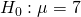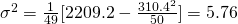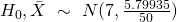# 2014 A-level H1 Mathematics (8864) Question 10 Suggested SolutionsAll solutions here are SUGGESTED. Mr. Teng will hold no liability for any errors. Comments are entirely personal opinions.

(i)
Let X denotes the length of a leaf in cm.Underapproximately by Central Limit Theorem since n is large.

From Graphing Calculator, p-value.

Thus we reject, and conclude with sufficient evidence at 5% level of significant that the mean length of the leaf is less than 7 cm.

(ii)

Unbiased estimate ofUnbiased estimate of(iii)Underapproximately by Central Limit Theorem since n is large.

From Graphing Calculator, p-value.

Sinceis rejected, p-valuesRequired set of values \$latex = \{ \alpha \in \mathbb{R}: 1.97 \le \alpha \le 100 \}

Not readable? Change text.